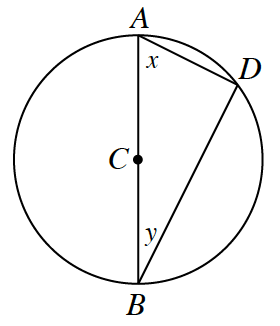### Home > INT2 > Chapter 10 > Lesson 10.2.3 > Problem10-80

10-80.Use the diagram of $⊙C$ at right to answer the questions below. Each part is a separate problem.

1. If $x = 28°$, what is $m\overarc { A D }$?

Recall that the measure of each inscribed angle is half the arc it intercepts.

What is the sum of interior angles for triangles?

1. If $AD = 5$ and $BD = 5\sqrt { 3 }$, what is the area of $⊙C$?

Knowing that $m∠D$ is $90°$, use the Pythagorean Theorem to solve for the $AB$.

Then from there, substitute the information you found in the equation for the area of a circle.

$25π$ units$^2$

2. If the radius of $⊙C$ is $8$ and if $m\overarc { B D }= 100°$ , what is $BD$?